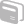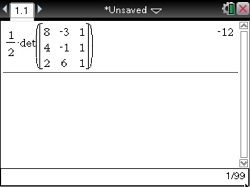# Activities

••• ##### Subject Area

• Math: Algebra II: Matrices

• ##### Author9-12

45 Minutes

• ##### Device
• TI-Nspire™ Navigator™
• TI-Nspire™
• TI-Nspire™ CAS
• ##### Software

TI-Nspire™
TI-Nspire™ CAS

3.0

## The Determinant of a Matrix#### Activity Overview

Calculate the determinant of a matrix and evaluate it to calculate the area of a triangle or quadrilateral.

#### Objectives

• Calculate the determinant of a matrix
• Evaluate a determinant to calculate the area of a triangle or quadrilateral in the plane, given the coordinates of its vertices